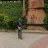# Intro to Measurement System Analysis (MSA) of Continuous Data – Part 3: Linearity

MSA

Tags:
1.This is the third in a series of articles about MSA. The focus of this article will be on measurement linearity.

Linearity is simply measurement bias throughout the entire range of the measurement device. For this reason bias and linearity are often combined into a single study.

A good calibration system will check the calibration of a measurement device at a minimum of three locations (both extremes and the middle of the measurement range). A thorough linearity study will check at least five locations (e.g., both extremes, and at 25%, 50% and 75% of the measurement range). Standards such as used in calibration should be used instead of actual parts unless the parts can be measured with less measurement variation with a different measurement device. Each standard is measured repeatedly at least ten times. All measurements must be made randomly to minimize the appraiser recalling previous results. The results can be analyzed using statistical software such as Minitab, or as shown.

• Calculate the bias for each individual measurement and the average bias for each reference standard (or part).

• Plot the individual and average biases on the y-axis of a scatter plot versus the values of the standards on the x-axis.

• Perform a regression analysis using the individual biases as the response and the reference values as the predictor variable.

• Plot the regression line and the 95% confidence limits for the regression line on the scatter plot.

• Plot the bias = 0 line for all reference values.

• Verify that the Bias = 0 line lies within the +/- 95% confidence limits of the regression line.

• The y – intercept and slope of the regression equation should each be approximately equal to zero. These values may be statistically evaluated if desired per the formulae in the AIAG MSA 4th edition manual. For practical purposes the graphical analysis is sufficient. The statistical analysis is only necessary for borderline cases.
Ideally, linearity will be statistically equal to zero. However, this will not always be the case. There are several possible scenarios:

• Constant bias – All measurements are offset by the same amount regardless of size. This is essentially a calibration issue. Calibrate the measurement device and repeat the linearity study.
• Non-constant, linearly increasing/decreasing bias –The bias either increases or decreases as the location within the measuring range increases. Possible causes: measurement scale is proportionally small/large (gage issue), thermal bias (thermal expansion/contraction is proportional to the size of the dimension), pressure bias (deflection under pressure is proportional to size), etc.
• Non-constant, nonlinear bias – The bias changes in a nonlinear fashion throughout the measurement range. Possible cause: measurement scale is nonlinear (gage issue, particularly with electronics), gage wear in one section of the measurement range, worn standards.
If the linearity is acceptable within the range actually used for measurement, the gage may be accepted for a specified range of measurement. This must be clearly noted on the gage and the practice documented in the appropriate quality procedures.

### Recent Reviews

1.judegu
4/5,
Good illustration on the Linearity